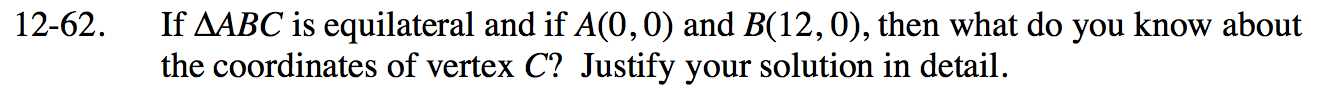### Home > INT2 > Chapter 12 > Lesson 12.2.1 > Problem12-62

12-62.

If ΔABC is equilateral and if A(0, 0) and B(12, 0), then what do you know about the coordinates of vertex C? Justify your solution in detail. 12-62 HW eTool (Desmos). Desmos Accessibility Homework Help ✎Use the eTool below to create your equilateral triangles.
Click the link at right for the full version of the eTool: Int2 12-62 HW eTool

Notice the position of the third vertex for both triangles. They both have an x-coordinate of 6.

Use the patterns you learned for special right triangles to find the height of the congruent equilateral triangles.

$\text{Vertex }C\text{ can be at }(6,6\sqrt{3})\text{ or }(6,-6\sqrt{3}).$